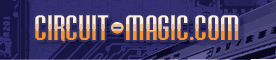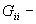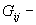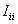Node Voltage analysis technique & circuit analysis using AKNM Circuit-Magic & Node VoltageCircuit analysis Tutorial
AKNM Circuit Magic- circuit analysis software

# Node Voltage Method

The node voltage method is deduced from the Kirchhoffĺs current law (KCL) and Ohmĺs laws

The following formulas are used to solve circuit.whereNode i conductivity is the sum of conductivities of all branches which connected with Node i.Node i to node j conductivity is the negative sum of conductivities of all branches which connect Node i with Node j..Node i - currentSample: Circuit Solution By Node Voltage

Step 1. Construct following circuit using circuit editor.

Step 2. Run solution by node voltage method.

Step 3. Correct following solution.

Electrical scheme

Initial variables

R1=10 ohms; R2=20 ohms; R3=25 ohms; R4=10 ohms; R5=10 ohms;

E1=10V; E2=15V;

J1=10A;

Solution

V1ĚG11+V2ĚG12+V3ĚG13=I11

V1ĚG21+V2ĚG22+V3ĚG23=I22

V1ĚG31+V2ĚG32+V3ĚG33=I33

Node 1 conductivity

G11=1/R2+1/R1+1/R4=0,25

Node 1 to Node 2 conductivity

G12=-1/R2=-0,05

G13=-1/R1=-0,1

G22=1/R2+1/R5=0,15

G23=-1/R5=-0,1

G33=1/R5+1/R1+1/R3=0,24

I11=-E2/R1=-1,5

I22=-J1=-10

I33=E2/R1-E1/R3=1,1

Linear equations

0,25V1-0,05V2-0,1V3=-1,5

-0,05V1+0,15V2-0,1V3=-10

-0,1V1-0,1V2+0,24V3=1,1

V1=-69,706

V2=-147,06

V3=-85,735

V4=0

Branch currents calculation using Ohmĺs Law

I1=(V1-V2)/R2=3,86765

I2=(V2-V3)/R5=-6,13235

I3=(V1-V3+E2)/R1=3,10294

I4=J1=10

I5=(V3-V4+E1)/R3=-3,02941

I6=(V1-V4)/R4=-6,97059